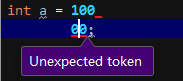``````int a = 100
00;
``````

6个回答

``````不知道你说的是什么编译器什么语言，如果是C#的话，那么标识符内部不能换行/空格。之间可以。

int abc = 123;

int
abc
=
123
;

int abc
= 123;

int
abc = 123;

int a
bc = 123;

3;

nowhere，是一个单词，但是如果可以随意拆分，是now here还是no where？那不搞不清楚了么
``````

int a = 0;

int b = 10;

``````int a =
10000;
``````

``````int a = 100
00;
//or
int a = 100     00;
``````

java语法无法解析100[空格][空格]00是什么东西,自然会报错，你可以在ide工具中查看错误信息。C语言里面换行要加\